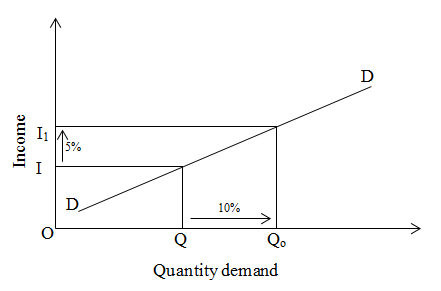# Income elasticity of demand greater than one

If the percentage change in quantity demand is greater than the percentage change in income is known as income elasticity of demand greater than one.
For example, change in demand by 10% due to change in income by 5%.

We can explain it by the help of given figure:On the above figure x and y-axis measures quantity demand and income respectively. DD is demand curve. In initial stage, income and demand is OI and OQ. When income is increased from I to I1 by 5% then demand is increased from Q to Q­1­ by 10%. This case is known is income elasticity of demand greater than one.